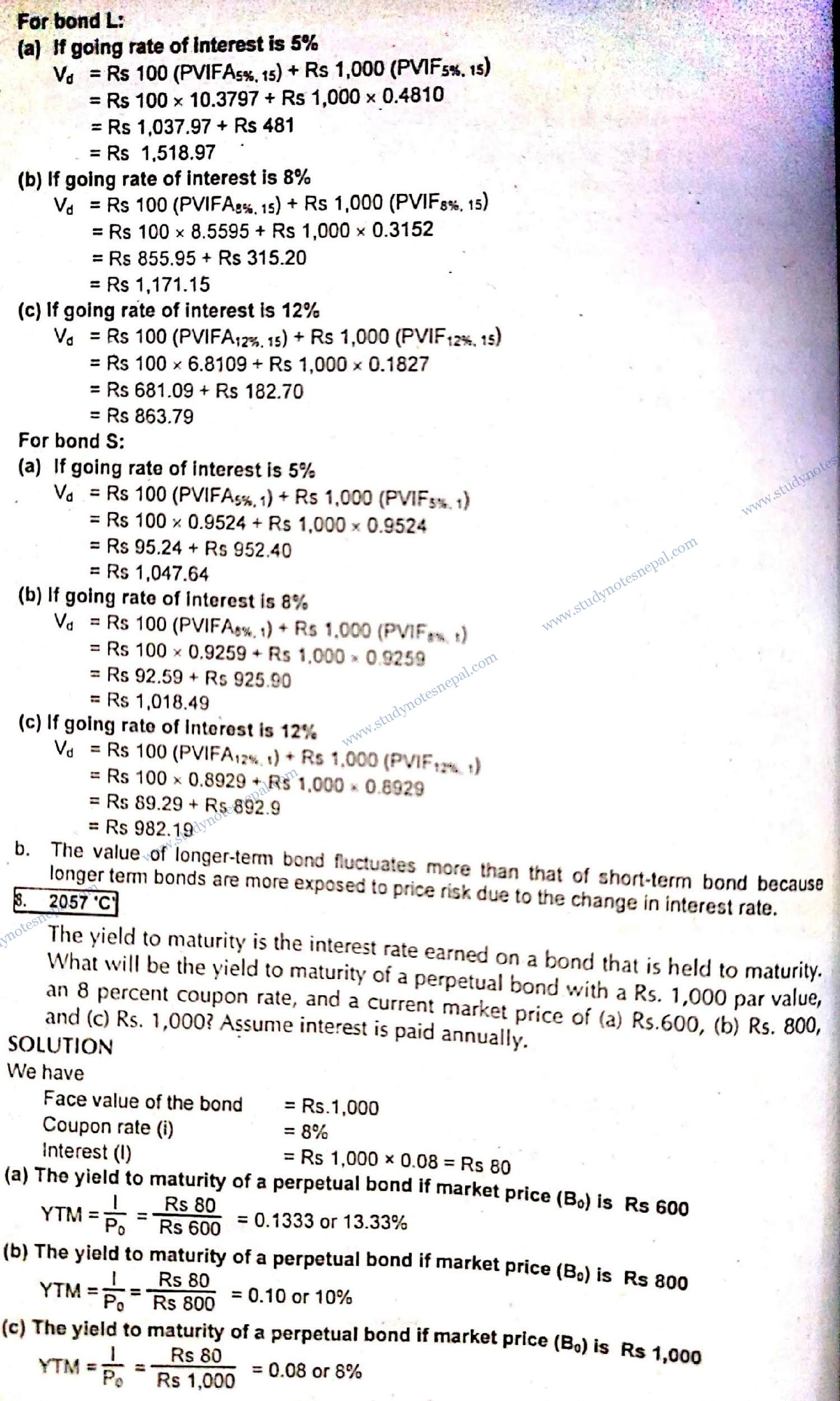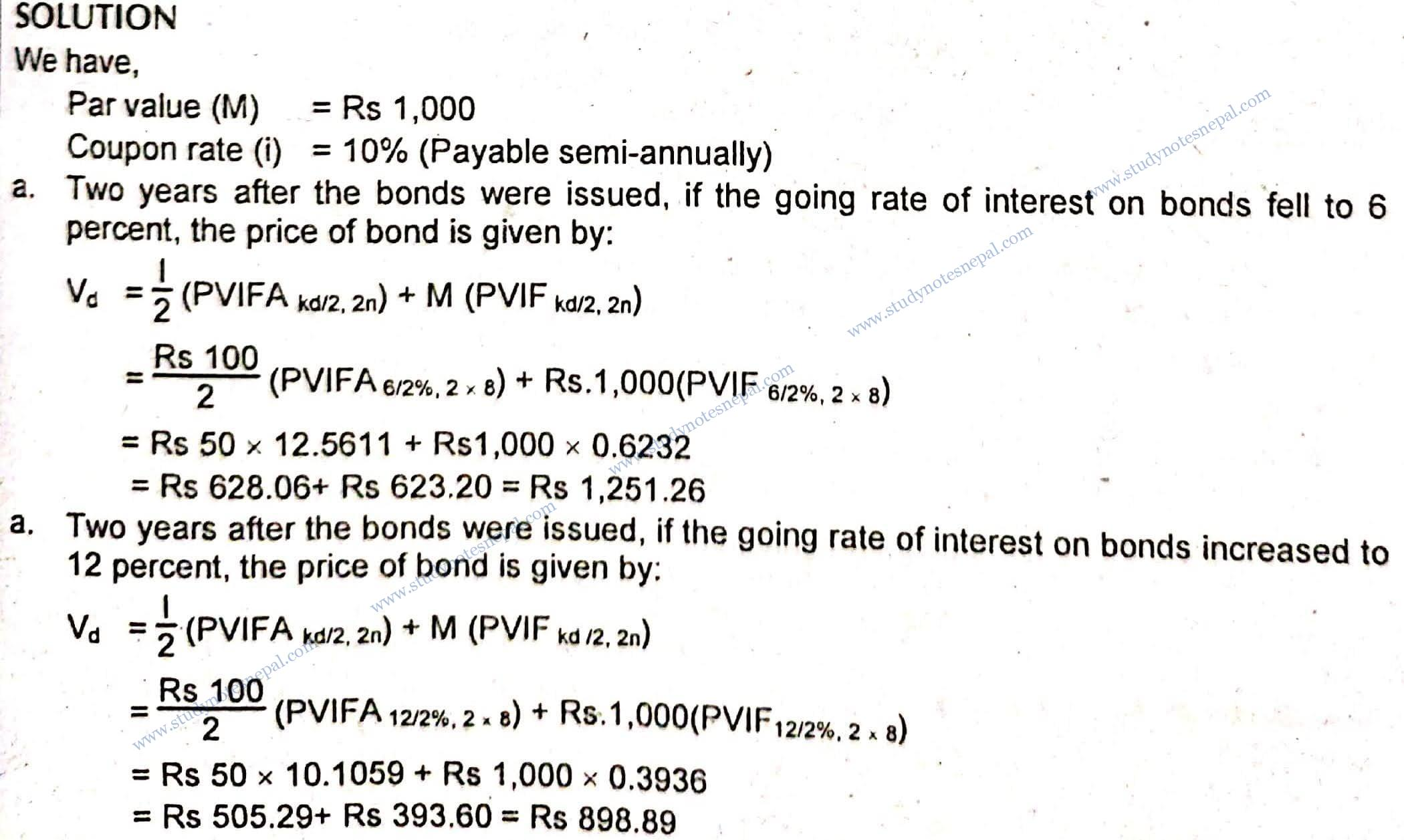##### 1. What are the key features of bond? Explain.

A bond is a long-term promissory note issued by corporations. It is called a promissory note because the issuer promises to pay a stated amount of interest regularly and repay the maturity value at the expiry of the maturity period. While issuing  bonds, the issuer prepares a legal document called indenture which is duly signed by the issuer and the trustee on behalf of the bondholders. It contains the terms and conditions of bond issues. The indenture specifies the following features of bond issue.

• Par value: The par value is the stated amount of face value per bond. It is usually set at Rs 1,000 per bond.
• Coupon rate: The coupon rate is the stated rate of interest to be paid on bond issue. This rate is calculated as a percentage on par value and paid annually, semi-annually, quarterly or monthly depending on the terms of bond issue.
• Maturity: Maturity is the stated expiry period of bond contract. At the expiry of the maturity period, bondholders are repaid the maturity value, which is equal to Rs 1,000 par value per bond, if other things are not stated.
• Call provision: Some bonds are issued with call provision which allows the issuer to call the bond for redemption at any time before the expiry of the maturity period. The bond containing this feature is called a callable bond.
• Conversion feature: Some bonds are also issued with conversion features. This feature allows the conversion of bonds into a stated number of shares of common or preferred stock at the option of the bondholders. A straight bond has no conversion feature.

#### Write Short Notes on

##### i) Bond valuation

Bond valuation is the process of determining the intrinsic value or fair value of a bond. The fair value of a bond is the total of the present value of periodic interest payment and the present value of the maturity value to be realized at the end of the maturity period. In the case of bonds carrying fixed maturity, there are two types of future cash flows associated with bond investment: one is the periodic coupon payment and the other is the maturity value. Such a bond is called a regular bond. Thus, the fair value of a bond carrying fixed maturity is the total of present values of these futures cash flows. It is given as follows:

Bo = I x [PVIFA,kd.n] + Mx [PVIF,kd.n]

In case of perpetual bonds, there is no stated maturity period and hence no stated maturity value. The only cash flows associated with such bonds are an infinite stream of coupon payment. Thus value of a perpetual bond is the present value of infinite stream of coupon payment and is given by:

B0 = I /kd

Note that in above equations, the value of bond also depends on the discount rate (kd) along with the associated future cash flows. This discount rate represents the bondholders required rate of return that is selected consistent to the level of risk associated with bond investment. For highly risky bonds, the required rate of return is also higher and as a result the value of bond declines with higher required rate of return.

##### ii) Yield to maturity

The yield to maturity (YTM) is the annualized rate of return that a bondholder can realize from bond investment if the bond is held until the maturity period. Technically speaking, YTM is the discount rate at which the present value of all future cash flows associated with bond investment remains equal to the current selling price of the bond. The YTM is the total annualized yield that contains both capital gain yield and the current yield from bond investment. Capital gain yield component of YTM results from the change in price of bond over time, whereas current yield is the interest return stated as a percentage of selling price of the bond. YTM calculation is based on the following assumptions:

• The bond is held until maturity and the bond issuer is not expected to call the bond before the expiry of the maturity period.
• Coupon interest income from bond investment each period is assumed to be reinvested for the remaining period until maturity to earn the YTM itself.
• The bond issuer pays all contractual payments as promised, that is, the issuer will not default on paying interest and repaying the maturity value.
##### iii) Changes in bond value over time

The value of a bond changes over time as it approaches the maturity period. On the basis of change in bond’s value over time, we can categorize two types of bonds- premium bond and discount bond. A bond is called a premium bond if it currently sells above par value. If the coupon rate on the bond is higher than market interest rate, the bond sells at premium. Other things remain the same, the value of premium bonds goes on declining as it approaches maturity. At maturity. the value of premium bond falls to Rs 1,000 par value.

On the other hand, a bond is said to be a discount bond if it sells below the par value. A bond sells at discount if it carries the coupon rate that is lower than the market interest rate. The value of discount bonds goes on increasing as it approaches maturity. At the expiry of the maturity period, the value of discount bond exactly increases to Rs 1,000 maturity value. Note that if the bond’s coupon rate is exactly equal to the market interest rate at the time of issue, the bond exactly sells at par value. This type of bond is called par bond. If the market interest rate remains constant until maturity, the value of par bond also remains constant.

##### iv) Bonds

Bonds are long-term promissory notes issued by corporations or governments. Bonds issued by corporations are called corporate bonds while those issued by the government are called Treasury bonds. They are called promissory notes because the issuer promises to pay a stated amount of interest regularly and repay the maturity value at the expiry of the maturity period. The stated rate of interest to be paid on bonds is called coupon rate. Coupon rate is expressed as a percentage on par value. The par value is the stated amount of face value per bond which is usually set at Rs 1,000 per bond. Generally, all bond issues have a fixed maturity date. At the expiry of the stated maturity date, the holders are repaid maturity value, which is usually equal to Rs 1,000 par value if other things are not stated. While issuing bonds, the issuer prepares a legal document called indenture. The indenture is duly signed by the issuer and the trustee on behalf of the bondholders. It contains the terms and conditions of bond issues. The indenture specifies call provision, conversion features, sinking fund provision and others.

## Numerical Problems

The Karnali Company has two bond issues outstanding. Both bonds pay Rs.100 annual interest plus Rs.1,000 at maturity. Bond L has a maturity of 15 years and Bond S a maturity of 1-year. What will be the value of each of these bonds when the going rate of interest is (a) 5 percent, (b) 8 percent, and (c) 12 percent? Assume that there is only one more interest payment to be made on Bond S. Why does the longer-term (15-year) bond fluctuate more when interest rates change than does the shorter-term band (1-year)?Suppose Tansen Textile Company sold an issue of bonds with a 10-year maturity, a Rs. 1,000 par value, a 10 percent coupon rate, and semiannual interest payments.

• Two years after the bonds were issued, the going rate of interest on bonds such as these fell to 6 percent. At what price would the bonds sell?
• Suppose that, 2 years after the initial offering the going interest rate had risen to 12 percent. At what price would the bonds sell?
• Suppose that the conditions in part (a) existed that is, interest rates fell to 6 percent 2 years after the issue date. Suppose further that the interest rate remained at 6 percent for the next 8 years. What would happen to the price of the Company’s bonds over time?• If the interest rate declines to 6 percent after the issue date and remains constant at this level for the remaining period until maturity, the price of bond declines and ultimately falls to Rs 1,000 maturity value at the end of maturity period.

Study Notes Nepal